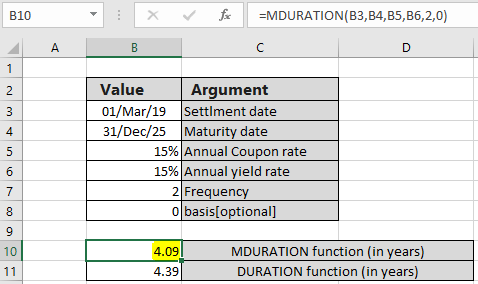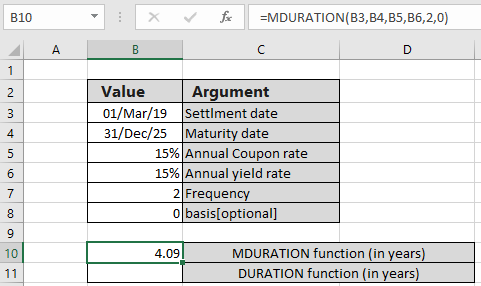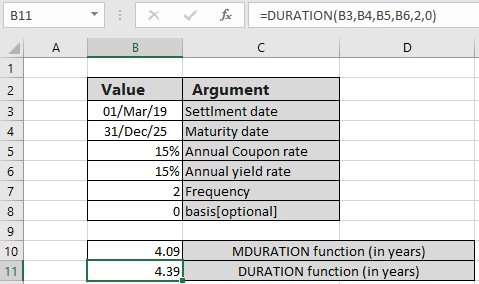# How to use the MDURATION function in ExcelIn this article, we will learn How to use the MDURATION function in Excel.

Scenario :

What is Macauley duration? Macauley duration is the weighted average time recorded to the maturity of cash flows for par value of \$100. Learn more mathematical formulas about macaulay duration here. We calculate the weighted average using the DURATION function in Excel. In contrast to Macaulay duration, modified duration (commonly known as MD) is a price sensitivity measure, defined as the percentage derivative of price with respect to yield for par value of \$100. In excel we can calculate the modified duration using the MDURATION function. The mathematical relation between the two can be shown as shown below.

Modified duration = macaulay duration / ( 1 + market yield/coupon payments per year)

MDURATION function in Excel

MDURATION function returns the modified duration in years for a security for assumed \$100 par value with some required terms. These required terms are given as argument to the function. Let's learn more about the argument in the syntax stated below.

MDURATION function syntax:

 =MDURATION( settlement, maturity , rate , yld , redemption , frequency, [basis])

Settlement : Settlement date of the security

Maturity : Maturity date of the security. The maturity date is the date when the security expires.

rate : The security's annual coupon rate

yld : The security's annual yield rate

frequency - payments per year (1 = annual, 2 = semi, 4= quarterly)

basis - [optional] Day count basis. Default is 0 and for see  the table below.

 Basis Day count basis 0 US (NASD) 30 / 360 1 actual / actual 2 actual / 360 3 actual / 365 4 European 30 / 360

Example :

All of these might be confusing to understand. Let's take an example and apply the function on the given terms on security. Here we have some terms which will be used as arguments in the function.

Use the MDURATION formula:

 =MDURATION( B3 , B4 , B5 , B6 , 2 , 0 )Here the function returns the 4.09 years for the stated terms on security \$100 assumed par value which is roughly 49 months.

Giving the same arguments to the duration function is stated below.

Use the DURATION formula:

 =DURATION( B3 , B4 , B5 , B6 , 2 , 0 )Here the function returns the 4.09 years for the stated terms on security \$100 assumed par value which is roughly 52 months.

You must be thinking what could these results be used for. MDURATION function returns the number of years

For example, assume bank A and bank B enter into an interest rate swap. The modified duration of the receiving leg of a swap is calculated as nine years and the modified duration of the paying leg is calculated as five years. The resulting modified duration of the interest rate swap is four years (9 years – 5 years).

Here are all the observational notes regarding using the formula.
Notes :

1. Excel stores dates as serial numbers and is used in calculation by the function. So it’s recommended to use dates as cell reference instead of giving direct argument to the function. Check the date values if the function returns #VALUE! Error.
2. The security settlement date is the date after the issue date when the security is traded to the buyer.
3. Arguments like settlement date, maturity date, frequency & basis are truncated to integers.
4. The function returns the #VALUE! Error if the settlement date & maturity date is not a valid date format.
5. The function returns the #NUM! Error if:
1. Payment Frequency argument is not one of the three (1, 2 or 4)
2. If the basis argument is either < 0 or  > 4.
3. If maturity date settlement value.
4. If coupon rate or yield rate argument is < 0
6. The function generates #VALUE! Error if arguments to the function is non - numeric.

Hope you understood How to use the MDURATION function in Excel. Find more articles about financial formulas and its related functions here. If you liked our blogs, share it with your friends on Facebook. And also you can follow us on Twitter and Facebook. We would love to hear from you, do let us know how we can improve, complement or innovate our work and make it better for you. Write to us at info@exceltip.com.

Related Articles

How to use the DURATION function in excel : The Excel DURATION function returns the annual duration of a security with periodic interest payments, calculated with the Macauley duration formula.

How to use the RECEIVED function in excel : calculates the amount which is received at maturity for a bond with an initial investment (security) and a discount rate, there are no periodic interest payments using the RECEIVED function in excel.

How to use the MIRR function in excel : returns the Modified interest rate of return for the financial data having Investment, finance rate & reinvestment_rate using the MIRR function in Excel.

Excel PV vs FV function : find Present Value using PV function and future value using FV function in Excel.

How to use the NPER function in excel : NPER function to calculate compounding periods in months in Excel.

How to use the PRICE function in excel : returns the price per \$100 face value of a security that pays periodic interest using the PRICE function in Excel.

Popular Articles:

How to use the IF Function in Excel : The IF statement in Excel checks the condition and returns a specific value if the condition is TRUE or returns another specific value if FALSE.

How to use the VLOOKUP Function in Exceln : This is one of the most used and popular functions of excel that is used to lookup value from different ranges and sheets.

How to Use SUMIF Function in Excel : This is another dashboard essential function. This helps you sum up values on specific conditions.

How to use the COUNTIF Function in Excel : Count values with conditions using this amazing function. You don't need to filter your data to count specific values. Countif function is essential to prepare your dashboard.

Terms and Conditions of use

The applications/code on this site are distributed as is and without warranties or liability. In no event shall the owner of the copyrights, or the authors of the applications/code be liable for any loss of profit, any problems or any damage resulting from the use or evaluation of the applications/code.